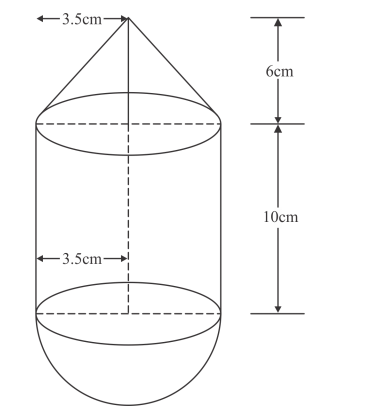# A solid is in the form of a right circular cylinder,Question:

A solid is in the form of a right circular cylinder, with a hemisphere at one end and a cone at the other end. The radius of the common base is 3.5 cm and the heights of the cylindrical and conical portions are 10 cm. and 6 cm, respectively. Find the total surface area of the solid. (Use π = 22/7)

Solution:

We have the following diagramFor cone, we have

$r=3.5 \mathrm{~cm}$

$h=6 \mathrm{~cm}$

$l=\sqrt{r^{2}+h^{2}}$

$=\sqrt{3.5^{2}+6^{2}}$

$=6.95 \mathrm{~cm}$

Curved surface area of the cone is given as

$S_{1}=\pi r l$

$=\frac{22}{7} \times 3.5 \times 6.946$

$=76.408 \mathrm{~cm}^{2}$

For cylindrical part, we have

$r=3.5 \mathrm{~cm}$

$h=10 \mathrm{~cm}$

Curved surface area of the cylinder is

$S_{2}=2 \pi r h$

$=2 \times \frac{22}{7} \times 3.5 \times 10$

$=220 \mathrm{~cm}^{2}$

The surface area of the hemisphere is

$S_{3}=2 \pi r^{2}$

$=2 \times \frac{22}{7} \times 3.5^{2}$

$=77 \mathrm{~cm}^{2}$

Total surface area of the solid is given by

$S=S_{1}+S_{2}+S_{3}$

$=76.408+220+77$

$=373.408 \mathrm{~cm}^{2}$

Hence the total surface area of the solid is $S=373.408 \mathrm{~cm}^{2}$